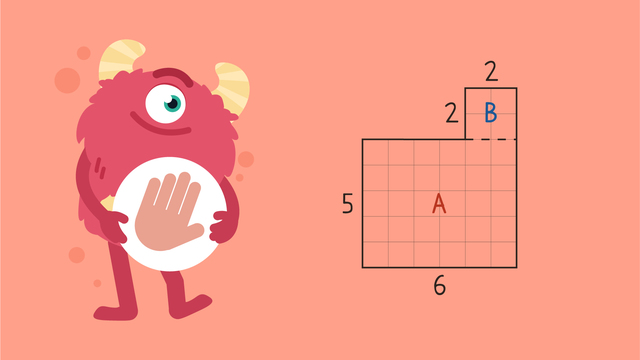# Area of Composite Shapes — Let's Practice!Rating

Ø 5.0 / 1 ratings
The authorsTeam Digital
Area of Composite Shapes — Let's Practice!
CCSS.MATH.CONTENT.3.MD.C.7.C CCSS.MATH.CONTENT.3.MD.C.7.D

## Basics on the topicArea of Composite Shapes — Let's Practice!

Today we are practicing area of composite shapes with Razzi! This video contains examples to help you further practice and grow confident in this topic.

### TranscriptArea of Composite Shapes — Let's Practice!

Razzi says get these items ready, because today we're going to find the area of composite shapes. It's time to begin! Find the area of this composite shape when we decompose it here. Pause the video to work on the problem, and press play when you are ready to see the solution! Shape A is six times five, which is thirty. Shape B is two times two, which is four. Thirty plus four equals thirty-four. Did you also get thirty-four square units? Let's tackle the next problem! Find the area of this composite shape when we decompose it here. Pause the video to work on the problem, and press play when you are ready to see the solution! Shape A is eight times two, which is sixteen. Shape B is three times four, which is twelve. Sixteen plus twelve equals twenty-eight. Did you also get twenty-eight square units? Let's tackle a tricky final problem! Find the area of this composite shape when we decompose it here and here. Pause the video to work on the problem, and press play when you are ready to see the solution! Shape A is nine times three, which is twenty-seven. Shape B is three times three, which is nine. Shape C is three times one, which is three. Twenty-seven plus nine plus three equals thirty-nine. Did you also get thirty-nine square units? Razzi had so much fun practicing with you today! See you next time!

## Area of Composite Shapes — Let's Practice! exercise

Would you like to apply the knowledge you’ve learned? You can review and practice it with the tasks for the video Area of Composite Shapes — Let's Practice!.
• ### Area of composite shapes.

Hints

The area is the inside of the entire shape.

Try counting the squares inside the shape.

Solution

The area of this shape is 22 unit squares.

• ### Find the areas of the shapes.

Hints

Sometimes it is easier to split the shape and then find the area. For example, this shape can be split in to two rectangles.

To find the area inside a composite shape, you can count the squares inside.

Solution

The composite shapes are shown here with their area in unit squares.

• ### What is the area?

Hints

Begin by splitting the shape into two rectangles.

Second, find the area of each rectangle separately.

Finally, don't forget to add the two parts together to get the total area.

Solution

To find the area of the composite shape.

• First partition the shape into two rectangles.
• Next, find the area of each rectangle.
• Finally, add the two areas together to find the total area.
• Area = 65 unit squares.

• ### What is the area of each shape?

Hints

Begin by splitting the shape into two rectangles.

When you have partitioned the shape, find the area of the two seperate rectangles, before adding them both together.

Solution

The area of the shapes is:

Shape 1 (blue): 30 unit squares

• 3 x 5 = 15
• 3 x 5 = 15
• 15 + 15 = 30

Shape 2 (green): 28 unit squares

• 2 x 10 = 20
• 2 x 4 = 8
• 20 + 8 = 28

Shape 3 (purple): 35 unit squares

• 2 x 10 = 20
• 3 x 5 =15
• 20 + 15 = 35

Shape 4 (red): 50 unit squares

• 3 x 12 = 36
• 2 x 7 =14
• 36 + 14 = 50

• ### What is the area of this shape?

Hints

How many squares are inside the entire shape?

Count across each row carefully, so that you don't miss any squares.

Solution

The area inside this composite shape is 17 unit squares.

• ### Use the area to find a side.

Hints

Begin by splitting the shape into two rectangles using the side lengths that have been given.

The area of shape b = 111 - area of shape a.

Use the given side to help find the missing side. The two blue sides are equal to the missing side.

Solution

To find the missing lenth shown here in yellow:

1) Find area b by solving the total area subtract area a. 111 - 45 = 66

2) Find side x by solving 66 ÷ 11 = 6

3) Add both vertical sides on the right (3 + 6) to find the vertical side on the left which is 9.